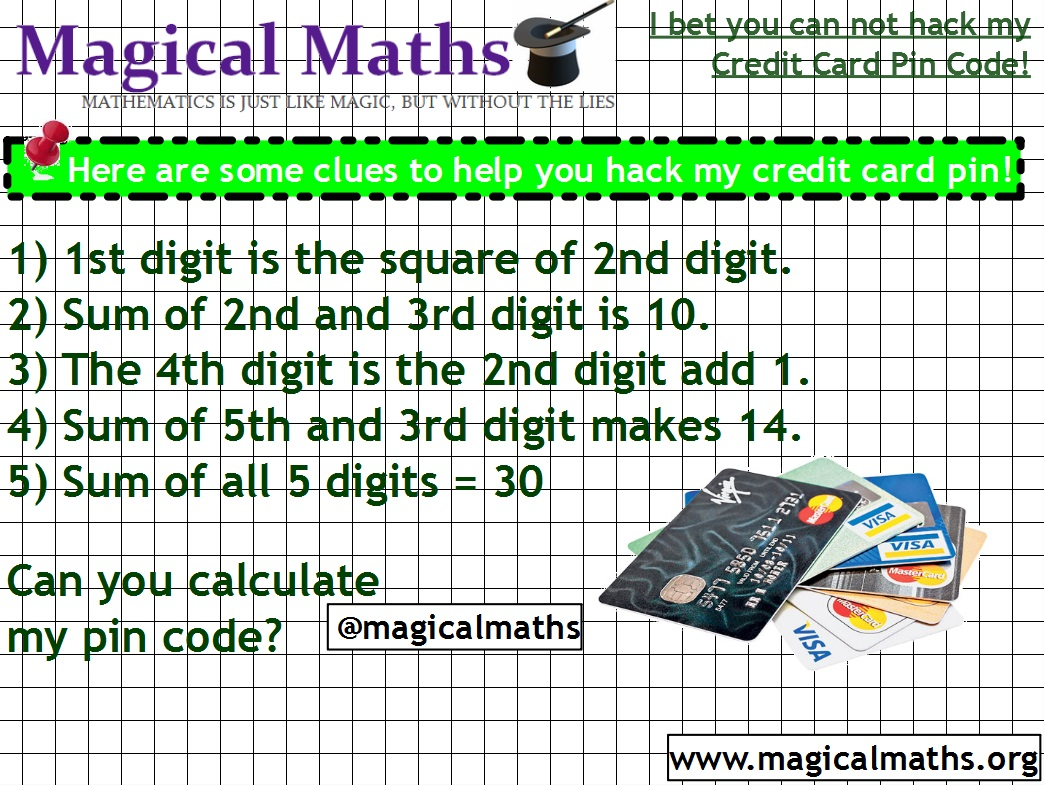How to hack credit cards using Mathematics! I bet you can not work out my credit card pin code!I bet you can not hack my credit card and work out my pin

Why not set your class a challenge to be able to work out the pin code to your credit card! This is a fun and engaging Mathematical starter that will get any pupil motivated to answer a great Math question. It is best used as a starter to a lesson, possibly on financial mathematics or a number lesson. Open your wallet and present to the class your credit card and tell them that the pupil who calculates your pin code first will get to keep the card (Obviously it is fake printed card). This normally increases the engagement and desire to hack the credit card pin code. Have fun!

Do not forget to check out all the other Magical Maths lesson starters available on the site.

Comments are FREE so please leave on below! So, what is my pin code? Answers below!

Financial Math lesson starterTagged with: , ,
One comment on “How to hack credit cards using Mathematics! I bet you can not work out my credit card pin code!”
1.John says:

replacing the 5 digits as a,b,c,d,e
a=b^2
b+c=10
d=b+1
e+c=14
a+b+c+d+e=30

Rearrange the last equation in terms of b:
b^2+b+(10-b)+(b+1)+(b+4)=30
simplifies to
b^2+2b=15

which yields two possible answers:
b=3 ,-5

Answer cannot be negative, therefore b=3
a=b^2=9
c=10-b=7
d=b+1=4
e=14-c=7

pin is therefore 93747